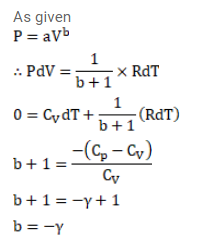# An ideal gas (c9/cv=????) is taken through a process inQuestion:

An ideal gas $\left(c_{9} / c_{\mathrm{V}}=\gamma\right)$ is taken through a process in which the pressure and the volume vary as $p=a V^{b}$.

Find the value of $b$ for which the specific heat capacity in the process is zero.

Solution: